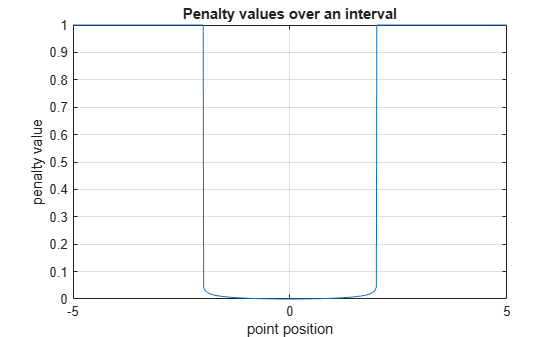# barrierPenalty

Logarithmic barrier penalty value for a point with respect to a bounded region

Since R2021b

## Syntax

``p = barrierPenalty(x,xmin,xmax)``
``p = barrierPenalty(___,maxValue,curvature)``

## Description

example

````p = barrierPenalty(x,xmin,xmax)` calculates the nonnegative (logarithmic barrier) penalty vector `p` for the point `x` with respect to the region bounded by `xmin` and `xmax`. `p` has the same dimension as `x`. This syntax uses the default values of `1` and `0.1` for the `maxValue` and `curvature` parameters of the barrier function, respectively.```

example

````p = barrierPenalty(___,maxValue,curvature)` specifies both the `maxValue` and `curvature` parameters of the barrier function. If `maxValue` is an empty matrix its default value is used. Likewise if `curvature` is an empty matrix or it is omitted, its default value is used.```

## Examples

collapse all

This example shows how to use the logarithmic `barrierPenalty` function to calculate the barrier penalty for a given point, with respect to a bounded region.

Calculate the penalty value for the point `0.1` within the interval [`-2`,`2`] using default values for the maximum value and curvature parameters.

`barrierPenalty(0.1,-2,2)`
```ans = 2.5031e-04 ```

Calculate the penalty value for the point `4` outside the interval [`-2`,`2`].

`barrierPenalty(4,-2,2)`
```ans = 1 ```

Calculate the penalty value for the point `4` outside the interval [`-2`,`2`], using a maximum value parameter of `5`.

`barrierPenalty(4,-2,2,5)`
```ans = 5 ```

Calculate the penalty value for the point 0.1 inside the interval [`-2`,`2`], using a curvature parameter of `0.5`.

`barrierPenalty(0.1,-2,2,5,0.5)`
```ans = 0.0013 ```

Calculate the penalty value for the point [`-2`,`0`,`4`] with respect to the box defined by [`0`,`1`], [`-1`,`1`], and [`-2`,`2`] along the x, y, and z dimensions, respectively, using the default value for maximum value and a curvature parameter of `0`.

`barrierPenalty([-2 0 4],[0 -1 -2],[1 1 2],1,0)`
```ans = 3×1 1 0 1 ```

Visualize Penalty Values for an Interval

Create a vector of 1001 equidistant points distributed between -`5` and `5`.

`x = -5:0.01:5;`

Calculate penalties for all the points in the vector, using the default value for the maximum value parameter and a value of `0.01` for the curvature parameter.

`p = barrierPenalty(x,-2,2,1,0.01);`

Plot the points and add grid, labels and title.

```plot(x,p) grid xlabel("point position"); ylabel("penalty value"); title("Penalty values over an interval");```## Input Arguments

collapse all

Point for which the penalty is calculated, specified as a numeric scalar, vector or matrix.

Example: `[0.5; 1.6]`

Lower bounds for `x`, specified as a numeric scalar, vector or matrix. To use the same minimum value for all elements in `x`, specify `xmin` as a scalar.

Example: `-1`

Upper bounds for `x`, specified as a numeric scalar, vector or matrix. To use the same maximum value for all elements in `x`, specify `xmax` as a scalar.

Example: `2`

Maximum value parameter of the barrier function, specified as a scalar.

Example: 2

Curvature parameter of the barrier function, specified as a scalar.

Example: 0.2

## Output Arguments

collapse all

Penalty value, returned as a vector of nonnegative elements. Each element `pi` depends on the position of `xi` with respect to the interval specified by `xmini` and `xmaxi`. The barrier penalty function returns the value

`$p\left(x\right)=\mathrm{min}\left({p}_{\mathrm{max}},C\left(\mathrm{log}\left(0.25{\left({x}_{\mathrm{max}}-{x}_{\mathrm{min}}\right)}^{2}\right)-\mathrm{log}\left(\left(x-{x}_{\mathrm{min}}\right)\left({x}_{\mathrm{max}}-{x}_{\mathrm{min}}\right)\right)\right)\right)$`

when xmin < x < xmax, and `maxValue` otherwise. Here, C is the argument `curvature`, and pmax is the argument `maxValue`. Note that for positive values of C the returned penalty value is always positive. If C is zero, then the returned penalty is zero inside the interval defined by the bounds, and pmax outside this interval. If x is multidimensional, then the calculation is applied independently on each dimension. Penalty functions are typically used to generate negative rewards when constraints are violated, such as in `generateRewardFunction`.

## Version History

Introduced in R2021b